+

# Preparing Balance Sheets

Author: Sophia Tutorial
##### Description:

Calculate the total assets, liabilities, and equity on a balance sheet that is prepared for a hypothetical business.

(more)### Developing Effective Teams

*No strings attached. This college course is 100% free and is worth 1 semester credit.

37 Sophia partners guarantee credit transfer.

299 Institutions have accepted or given pre-approval for credit transfer.

* The American Council on Education's College Credit Recommendation Service (ACE Credit®) has evaluated and recommended college credit for 32 of Sophia’s online courses. Many different colleges and universities consider ACE CREDIT recommendations in determining the applicability to their course and degree programs.

Tutorial
what's covered
This tutorial will cover how to prepare a balance sheet.

Our discussion breaks down as follows:

1. Balance Sheet: A Review
2. Balance Sheet: Example

## 1. Balance Sheet: A Review

In review, a balance sheet is a financial statement that provides information about the assets, liabilities, and equity of a business at a given time.

The balance sheet is the business's position. It captures a moment in time, rather than spanning a period, and it is the only financial statement that is prepared at a specific date.

The balance sheet details a business's resources, meaning assets, or the resources owned or available, liabilities owed to others, and the net difference on a cumulative basis, which is the equity.

The accounting equation is a fundamental premise in accounting, which states that a company's assets will be equal to the sum of its liabilities and its equity. This also happens to be the balance sheet formula; the accounting equation and the balance sheet formula are the same.

formula
Accounting Equation/Balance Sheet Formula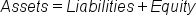Keep in mind, this formula will guide us as we walk through an example of preparing a balance sheet, in the next section.

## 2. Balance Sheet: Example

Let's get started on preparing our balance sheet. The starting point for preparing the balance sheet is the adjusted trial balance, which we can pull from the trial balance worksheet, below.

As you can see, we've already gone through the steps of preparing our trial balance, making our adjustments, and preparing the adjusted trial balance, which is what we used to build the financial statements. Note the detail of all of the ledger accounts in the adjusted trial balance.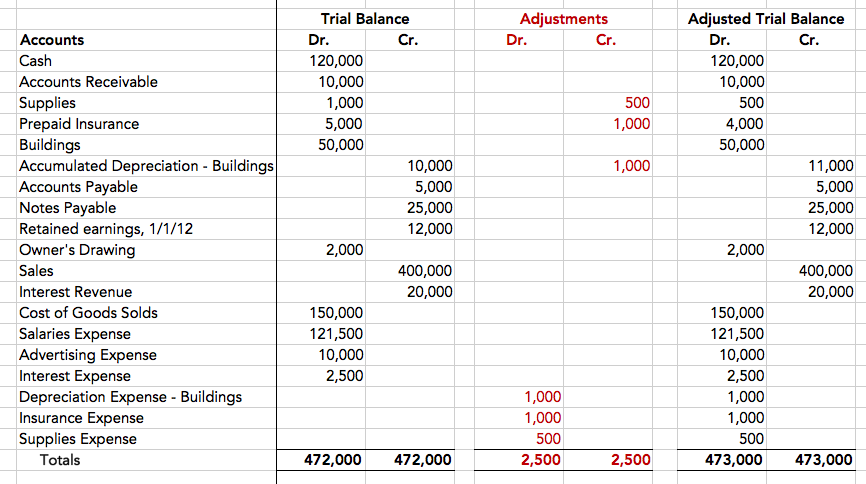However, in order to prepare our balance sheet, we also need to have our income statement information, because we need to know how much net income there is for the year and plug this information into our equity. Therefore, here is all the raw information that we need to prepare the balance sheet--the adjusted trial balance, as well as the income statement.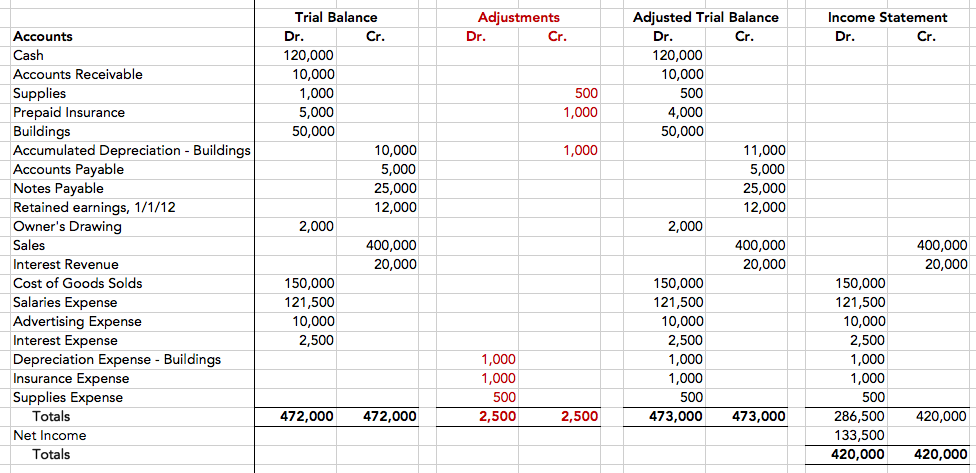Now that we've gathered the information that we need from the trial balance worksheet, let's start pulling it into our balance sheet. Remember, a balance sheet always starts with a header, which includes the business name, "Balance Sheet," and "As of" a specific date, which, in this case, is December 31, 2012.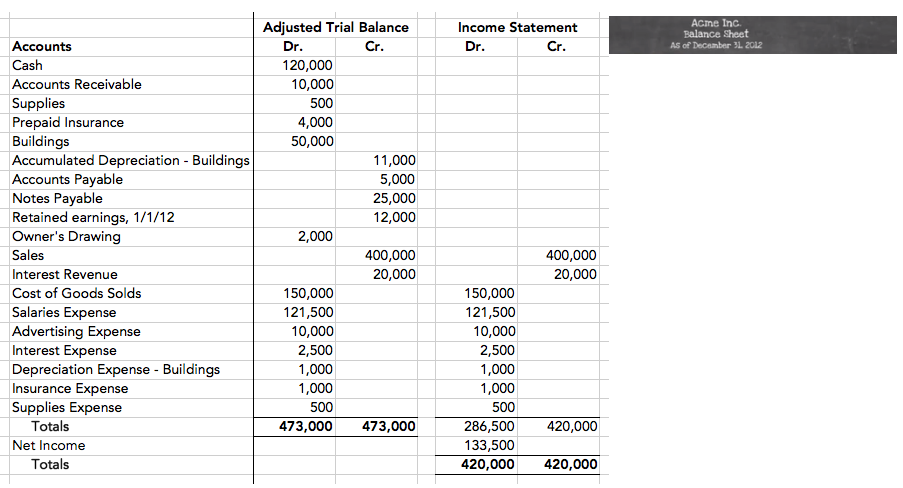Let's refer back to our adjusted trial balance information, below. Here are all of our permanent accounts, which represent all of our assets, liabilities, and equity. These are the permanent accounts that we need to prepare the balance sheet.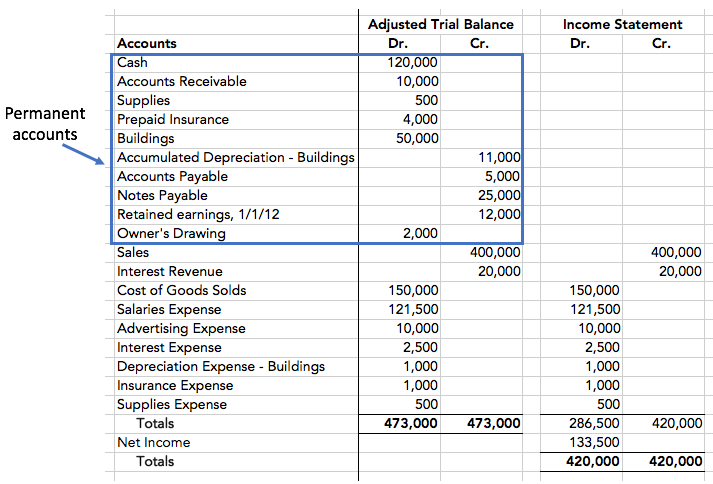We'll start with the current assets--cash, accounts receivable, supplies, and prepaid insurance. Then, we will input the long-term assets, which, in this case, are buildings and accumulated depreciation, which total \$39,000. Adding together our current assets and long-term assets, we arrive at total assets in the amount of \$173,500.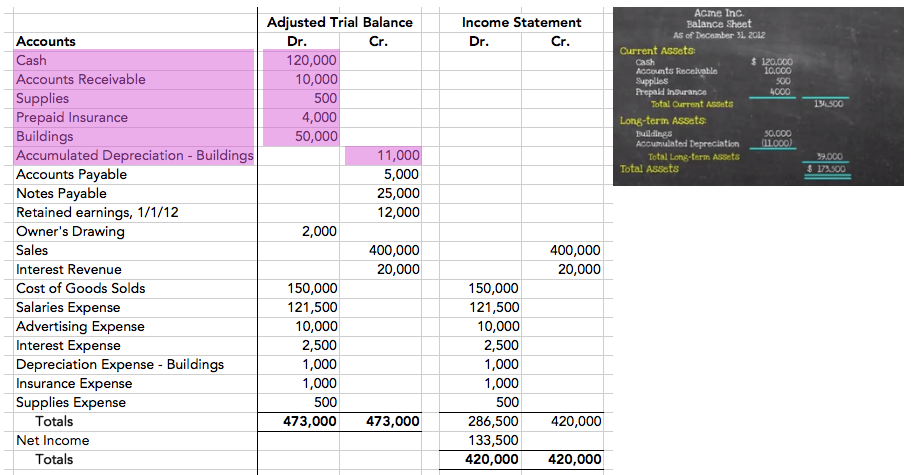Now, recall the accounting equation/balance sheet formula: Assets equal liabilities plus equity. Therefore, the next step is to input our liabilities. Short-term liabilities are accounts payable, and long-term liabilities are notes payable, which sum to total liabilities of \$30,000.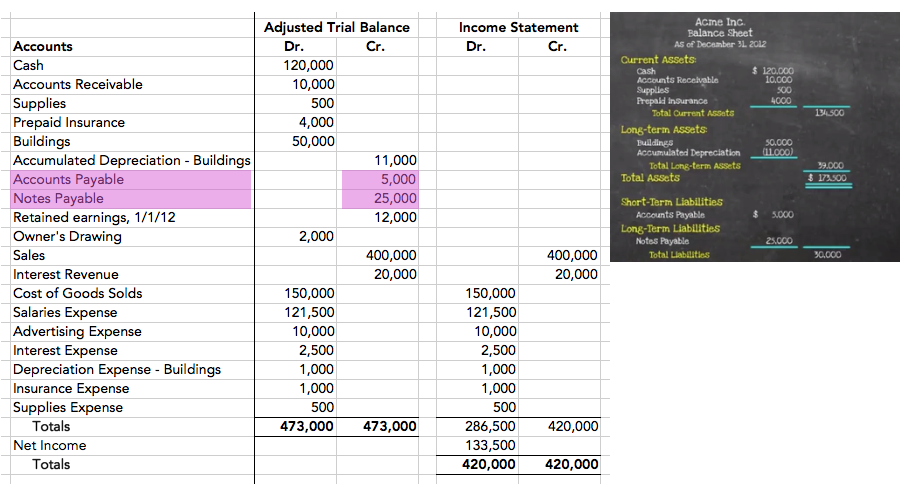The final piece is equity, starting with retained earnings of \$12,000, pulled from the adjusted trial balance. The next equity detail comes from the income statement in the form of net income for the period, in the amount of \$133,500. We also have owner drawings, from the adjusted trial balance, of \$2,000; we need to subtract those out for our total equity.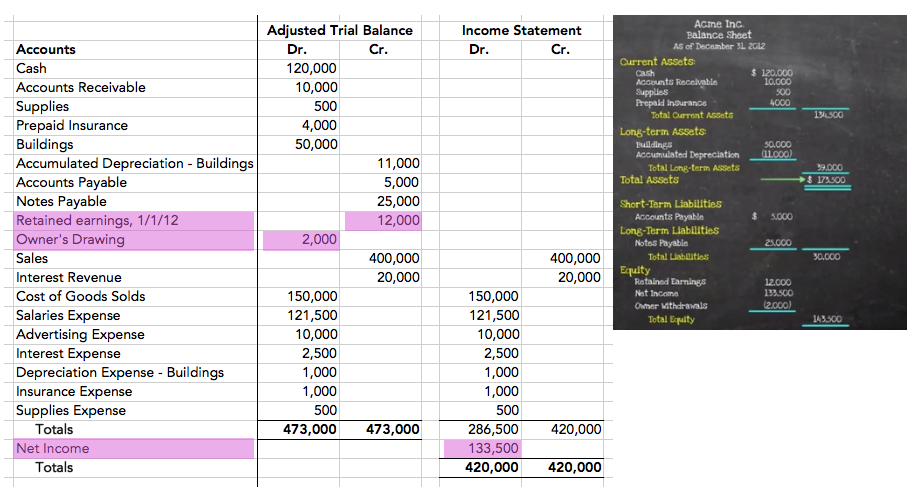Finally, we need to add total liabilities and equity. As you can see, we have total assets of \$173,500, and we also have total liabilities and equity of \$173,500. They are equal. Our accounting equation balances, as does our balance sheet formula.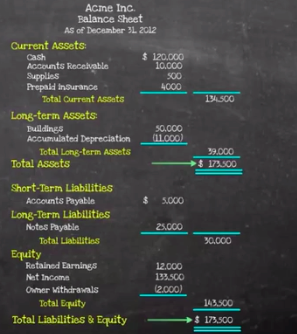summary
Today we covered a review of the balance sheet, which is a financial statement that provides information about the assets, liabilities, and equity of a business at a given time. We also explored a comprehensive example of preparing a balance sheet, making sure that at the end, our total assets were equal to the sum of our liabilities and equity.

Source: Adapted from Sophia instructor Evan McLaughlin.

Rating